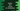# Python program to find the volume of a tetrahedron## Python program to find the Volume of a Tetrahedron:

In this post, we will learn how to find the volume of a regular Tetrahedron in python. Tetrahedron is a triangular pyramid. It has four triangular faces and six edges.

If its faces are equilateral triangles, it is called a regular tetrahedron. All edges are of equal lengths and all faces have same size and shapes.

### Volume of a Tetrahedron:

The volume of a regular tetrahedron is defined as below:

``a3/(6√2)``

Where, a is the length of its sides. The length of all edges are equal since it is a regular tetrahedron.

So, if we can read the length of a side as input from the user, we can calculate its volume.

### Python program:

We will write one python program that will take the length of the edges of the regular tetrahedron as input from the user and print the volume.

Below is the complete python program:

``````import math

def find_volume(a):
return (a ** 3 / (6 * math.sqrt(2)))

if __name__ == '__main__':
side_length = float(input('Enter the length of the sides: '))

print('Volume: {:.2f}\$'.format(find_volume(side_length)))``````

Here,

• find_volume method is used to find the volume of a regular tetrahedron.
• This method takes the length of the edges as the parameter and returns the volume.

### Sample output:

If you run this program, it will print outputs as like below:

``````Enter the length of the sides: 5
Volume: 14.73\$

Enter the length of the sides: 5.23
Volume: 16.86\$

Enter the length of the sides: 11.24
Volume: 167.35\$``````